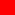## KALYAN CITY LIFE

Sharing Wisdom and Vivid Memories of Life

# Equilibrium Price Determination Under Perfect Competition

##Price Determination Under Perfect Competition ↓

In prefect competition, price is determined by the market forces of demand and supply. All buyers and sellers are price takers and not price makers. Buyer represents demand side in the market. Every rational buyer aims at maximising his satisfaction by purchasing more at lower price and lower at higher price. This is called demand behaviour of buyer i.e. Law of Demand.

Seller represents supply side in the market. Every rational seller aims at maximizing his profits by selling more at higher price and lesser at lower price. This is called supply behaviour of seller i.e. Law of supply.

But at a common price, buyer is ready to demand a particular quantity of goods and seller is also ready to supply exactly the same quantity of goods to buyer, such common price is called 'Equilibrium Price' and such quantity is called 'Equilibrium Quantity'.

"Equilibrium Price is a price which equates both demand and supply".

##Table - Sample Demand and Supply Schedules ↓

It is the price at which total demand is exactly equal to total supply. Graphically it is the point where DD curve and SS curve intersect each other.

##Graph - Equilibrium Price Determination ↓

In the above graphical diagram, the following points have been observed :-

1. On X axis, quantity demand and supplied per week has been given and on Y axis, price has been given.
2. Buyers are purchasing more at lower price and vice versa. This negative relationship is shown by downward sloping DD curve.
3. Sellers are selling more at higher price and vice versa. This positive relationship is shown by upward sloping SS curve.
4. Rs. 30 is that price at which demand equates supply (300 units). So, Rs. 30 is an equilibrium price and 300 units is an equilibrium quantity.
5. Suppose, price fails to Rs. 20/-, So this results into increase in demand (as per Law of Demand) and decrease in supply (as per Law of Supply). Since DD > SS, i.e. because of low supply, sellers will be dominant and competition will be among buyers, this leads to rise in price level. (i.e. from Rs. 20 to Rs. 30) Again price will come back at original level i.e. equilibrium price (Rs. 30).
6. Suppose, supply exceeds demand (DD < SS) now buyers become dominant and competition will be among sellers. This leads to downfall in price. (i.e. from Rs. 40 to Rs.30). Again price will come back to original level. i.e. equilibrium price (Rs. 30).
7. Such automatic adjustment by demand and supply forces will keep single price in market.

## Sitemap of Kalyan City Life Blog

### 1 Comment:

1. Anonymous said...

Simple to comprehend and easy to follow